Register for Maths, Science, English, Reasoning Olympiad Exams Register here | Book Free Demo for Live Olympiad Classes here | Check Olympiad Exam Dates here | Buy Practice Papers for IMO, IOM, HEO, IOEL etc here | Login here to participate in all India free mock test on every Saturday

# Roman Numerals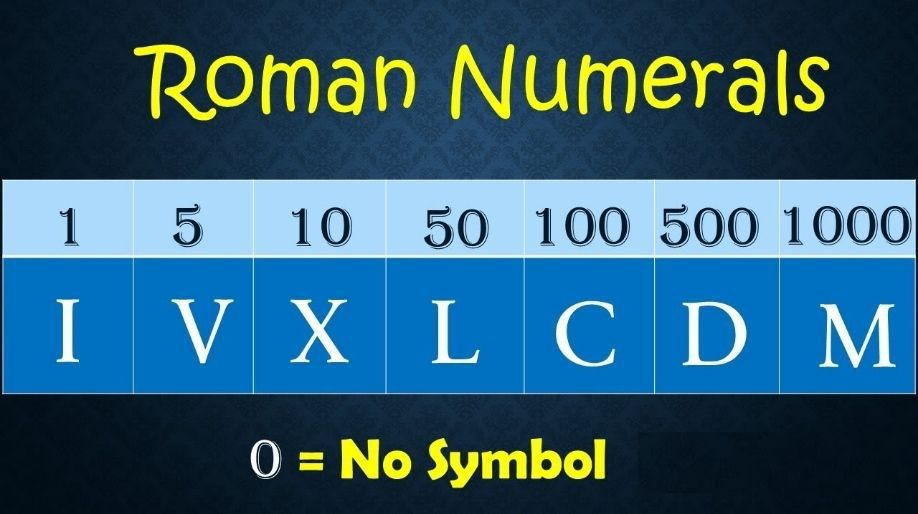Roman Numerals

Roman numerals were originated in ancient Rome to calculate the numbers and values.

The number system we are using is Hindu-Arabic Numeral System.

Once you remember these seven symbols , reading roman numerals is very easy.

Have a look on roman numerals from 1-10.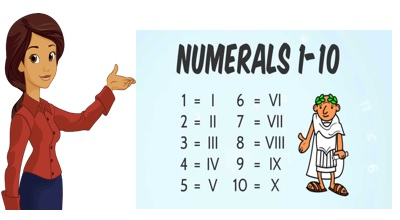If a symbol is repeated, its value increased as many times as it occurs, but the same symbol cannot be used more than 3 times.

Example: I = 1, II=2, III = 3

X = 10, XX = 20, XXX = 30

Did you observe that we cannot use the same symbol more than 3 times?

There is no numeral value for zero.

So, there are seven basic symbols that we use to read and write roman numerals: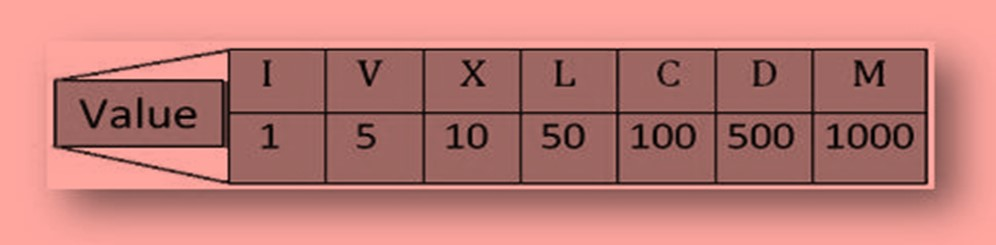How to Write Other Numbers?

If a symbol of lower value is written to the left of greater value symbol, then its value is subtracted from the value of the greater symbol.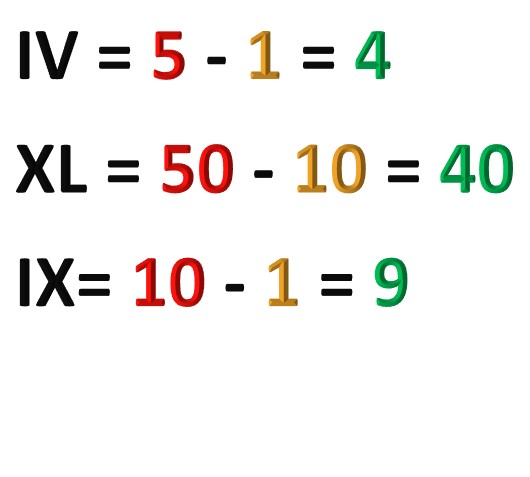If a symbol of greater value is written to the left of lower value symbol, then their values are added.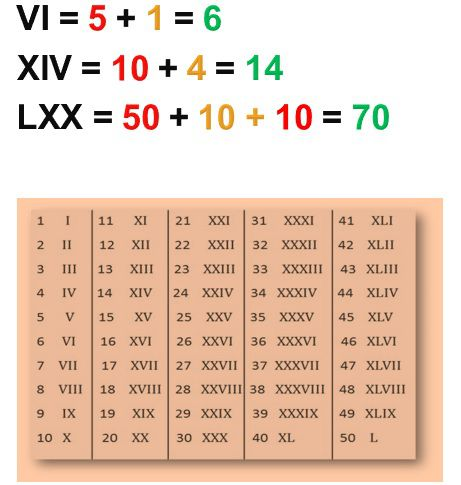Practice Exercise

Write the Roman Numerals for following numbers:

• 6
• 28
• 39
• 50
• 64

Answers: 1) VI   2) XXVIII   3) XXXIX   4) L   5) LXIV

Write the numbers for following:

• VII
• XIV
• XXIX
• XLIX
• C

Answers: 1) 7   2) 14   3) 29   4) 49   5) 100

Match the Roman Numerals with Numbers:

 V 60 XII 30 LX 104 CIV 5 XXX 12

 V 5 XII 12 LX 60 CIV 104 XXX 30

Recap

• Roman numerals are represented with symbols.
• There is no roman numeral for zero.
• We cannot use same symbol more than 3 times.
• Important symbols: V=5, X=10, L=50, C=100, D=500, M=1000

## Quiz for Roman Numerals

 Q.1 Add XII and XIV a) 20 b) 24 c) 26 d) 29
 Q.2 Find the difference between XIII and VIII a) 4 b) 5 c) 21 d) 15
 Q.3 Which is greater in value XIV or XVI? a) XIV b) XVI c) Incorrect question d) Both are equal
 Q.4 What would be your answer in Roman Numerals if someone ask you to write how many minutes are there in an hour? a) CX b) LX c) XL d) XC
 Q.5 Divide 30 by 2 and answer in roman numeral. (your answer should be the quotient) a) XV b) VX c) VV d) VVV
 Q.6 Riya had 6 books and her friend Rahul gifted her 5 more, then what is the count of her books in Roman Numeral? a) XI b) VIV c) VVI d) IX
 Q.7 What possibly can be the answer if I ask you to add XXII and III? a) 19 b) 25 c) 22 d) 3
 Q.8 What time is 3'o clock? Answer in roman numbers. a) III b) IVI c) X11 d) II
 Q.9 Anita's mother is 40 years old and Anita is 15 years old. What is the difference in their ages? a) VXX b) XVX c) XXV d) VVVVV
 Q.10 LLXII equals a) 222 b) 1522 c) 112 d) 211